# Linear Programming Model Formulation

In fact, the most difficult problem in the application of management science is formulation.

Therefore, it is important to consider linear programming model formulation before launching into the details of linear programming (LP) solution.

Model formulation is the process of transforming a real word decision problem into an operations research model. In the sections that follow, we give several Lilliputian examples so that you can acquire some experience.

All the examples that we provide in the following sections are of static models, because they deal with decisions that occur only within a single time period.

"Model formulation is partially an art." -Vinay Chhabra & Manish Dewan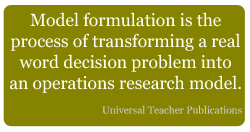In product mix selection, the decision-maker strives to determine the combination of the products, which will maximize the profit without violating the resource constraints. In the instance below, the objective is to maximize the profit. In the subsequent examples, you will see other objectives, such as minimum cost.

## Examples of Linear Programming Model Formulation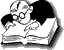Example 1

Universal Corporation manufactures two products- P1 and P2. The profit per unit of the two products is Rs. 50 and Rs. 60 respectively. Both the products require processing in three machines. The following table indicates the available machine hours per week and the time required on each machine for one unit of P1 and P2. Formulate this product mix problem in the linear programming form.

 Machine Product Available Time (in machine hours per week) P1 P2 1 2 1 300 2 3 4 509 3 4 7 812 Profit Rs. 50 Rs. 60

Solution.

Let x1 and x2 be the amounts manufactured of products P1 and P2 respectively. The objective here is to maximize the profit, which is given by the linear function

Maximize z = 50x1 + 60x2

Since one unit of product P1 requires two hours of processing in machine 1, while the corresponding requirement of P2 is one hour, the first constraint can be expressed as
2x1 + x2 ≤ 300

Similarly, constraints corresponding to machine 2 and machine 3 are
3x1 + 4x2 ≤ 509
4x1 + 7x2 ≤ 812

In addition, there cannot be any negative production that may be stated algebraically as
x1 ≥ 0, x2 ≥ 0

A variable that is also allowed to assume negative values is said to be unrestricted in sign.

The problem can now be stated in the standard linear programming form as

Maximize z = 50x1 + 60x2

subject to

2x1 + x2 ≤ 300
3x1 + 4x2 ≤ 509
4x1 + 7x2 ≤ 812

x1 ≥ 0, x2 ≥ 0

### Key Assumptions in Mathematical LP Formulation

Additivity. The total amounts of each input and the corresponding profit are the sums of the inputs and profit for each individual process. For example, one unit of Product 1 and one unit of Product 2 require 3 (2 + 1) hours of processing in machine 1, 7 (3 + 4) hours of processing in machine 2, 11 (4 + 7) hours of processing in machine 3, and yield Rs.110 (50 + 60) profit.

Divisibility. The activity levels are allowed to assume fractional values as well as integer values. For example, we admit the possibility of x1 = 509/3 or 169.67, x2 = 509/4 or 127.25.

Proportionality. The total amounts of each input and the associated profit are directly proportional to the level of output.

Doubling (or tripling) the production of a product will exactly double (or triple) the profit and required resources.

For example, to manufacture 1 unit of product 1 (x1 = 1), we require 2 hours of processing in machine 1, 3 hours of processing in machine 2, 4 hours of processing in machine 3, and the profit is Rs.50. By the same token, to manufacture 10 units of product 1 (x1 = 10), we require 20 hours of processing in machine 1, 30 hours of processing in machine 2, 40 hours of processing in machine 3, and the profit is Rs.500.

For all the examples in the subsequent sections of this chapter, we assume these assumptions to hold good.

##### Packaging ProblemExample 2

The Best Stuffing Company manufactures two types of packing tins- round & flat. Major production facilities involved are cutting and joining. The cutting department can process 200 round tins or 400 flat tins per hour. The joining department can process 400 round tins or 200 flat tins per hour. If the contribution towards profit for a round tin is the same as that of a flat tin, what is the optimal production level? Formulate a linear programming model for this problem (LPP).

Solution.

Let
x1 = number of round tins per hour
x2 = number of flat tins per hour

Since the contribution towards profit is identical for both the products, the objective function can be expressed as x1 + x2. Hence, the problem can be formulated as

Maximize Z = x1 + x2

subject to

(1/200)x1 + (1/400)x2 ≤ 1
(1/400)x1 + (1/200)x2 ≤ 1

x1 ≥ 0, x2 ≥ 0

i.e., 2x1 + x2 ≤ 400
x1 + 2x2 ≤ 400

x1 ≥ 0, x2 ≥ 0

By studying the above linear programming model examples and answering the exercises given at the end of this chapter, you will get good experience of LP formulation.

•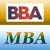MBA & BBA
•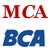BCA & MCA
•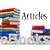Ebooks & Articles
•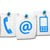Contact us